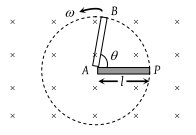A copper rod of length l is rotated about one end perpendicular to the magnetic field B with constant angular velocity ω. The induced e.m.f. between the two ends is

(1) $\frac{1}{2}B\omega {l}^{2}$

(2) $\frac{3}{4}B\omega {l}^{2}$

(3) $B\omega {l}^{2}$

(4) $2B\omega {l}^{2}$

Concept Videos :-

#6 | Motional EMF (Induced Electric Field)
#7 | Problem Set1: Motional EM
#8 | Problem Set2: Motional EM
#9 | Problem Set3: Motional EMF
#10 | Problem Set4: Motional EMF
#11 | Equivalent Circuit Diagrams

Concept Questions :-

Motional emf

(1) If in time t. the rod turns by an angle θ, the area generated by the rotation of rod will be

$=\frac{1}{2}l×l\theta$ $=\frac{1}{2}{l}^{2}\theta$So the flux linked with the area generated by the rotation of rod

$\varphi =B\text{\hspace{0.17em}}\left(\frac{1}{2}{l}^{2}\theta \right)\mathrm{cos}0=\frac{1}{2}B{l}^{2}\theta =\frac{1}{2}B{l}^{2}\omega \text{\hspace{0.17em}}t$

And so $e=\frac{d\varphi }{dt}=\frac{d}{dt}\left(\frac{1}{2}B{l}^{2}\omega t\right)=\frac{1}{2}B{l}^{2}\omega$

Difficulty Level:

• 88%
• 4%
• 6%
• 4%